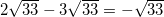## Example Questions

### Example Question #1 : How To Subtract Square Roots

Which of the following is equivalent to the expression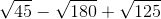?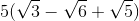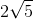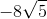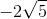Explanation:

The most difficult element of this problem is being able to correctly simplify each of the square roots.  This means starting with our first contestant,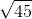.  There are several different methods for simplifying square roots, each with their own benefits.  Furthermore, some calculators permissible on the ACT can actually do it for you (might be worth looking into).  But for those of us not so fortunate, here is one particular method, which utilizes what is called a factor tree.

Start by writing the number inside the square root, 45 in our case.  And find two factors of (numbers that multiplied together equal) 45 other than 1 and the number itself.  In our case there are two possibilities:  9 and 5 or 3 and 15.  Both will work, but we will go with the latter pair. Look at each of the two factors to see if the same process can be repeated.  Looking at 3, we realize it is prime, meaning no additional factors exist.  Therefore, we leave the 3 as is.  However, the 15 can be further broken up into the factors 5 and 3, which we can illustrate as shown. We then attempt to repeat the process with the next tier of numbers, but we soon realize that both 5 and 3 are prime.  Therefore, we can go no further, and our factor tree is finished.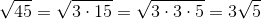But what do we do now?  We look for pairs. For each pair of numbers we have under the radical, we put one number on the outside of the square root.  That means one 3 goes outside the square root.  For each under the radical without a pair, we put that number inside the square root, meaning one 5 goes inside the square root.  This gives a final answer of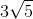.

We then repeat the process with our second contestant,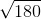.  A factor tree for 180 could take several different paths, but any correct one (including the example below) should end with the same numbers under the radical. Though perhaps not in the same order, the numbers under the radical should include two 2s, two 3s, and one 5. We see that a pair of 2s and a pair of 3s should give us one 2 and one 3 on the outside of the square root, while a pairless 5 should go on the inside.  Any time multiple numbers end up on either the inside or outside, we simply multiple those numbers.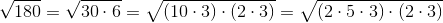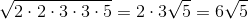Therefore, we get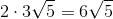.

We then complete the process one more time with our final contestant, 125.  Doing so invariably gives the following factor tree.

The problem in this case is that we don't have a pair but a trio of 5s.  In this case, we simply pair two of them, leaving one to be the odd five out.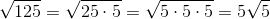Our final answer for this contestant then is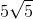.

Substituting our simplified square roots for the originals gives us the new expression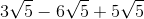.  From here it is best to think of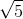like an apple.  In the first term I have 3 "apples".  I then subtract, or take away, 6 "apples".  Finally, I add back 5 "apples".  How many apples do I have?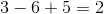.  I have 2 apples, or in other words.

### Example Question #2 : How To Subtract Square Roots

What is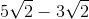in its simplest form?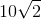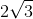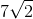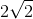Explanation:

Sinceandare both followed by the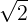, they can be subtracted normally. Thedoes not change, as it cannot be simplified.

### Example Question #3 : How To Subtract Square Roots

Simplify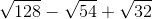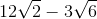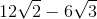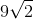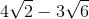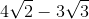Explanation:

Find the largest factors of 128, 54, and 32 that are perfect squares. For 128, the factors are 64 and 2, thus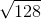=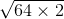. For 54, the factors are 27 and 2, thus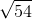=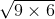. For 32, the factors are 16 and 2, thus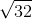=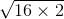. Simplifying these terms, leads to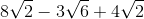. Combining like terms results in the final answer of.

### Example Question #4 : How To Subtract Square Roots

Simplify the following: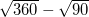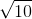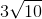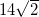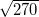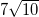Explanation:

Begin simplifying by breaking apart the square roots in question. Thus, you know: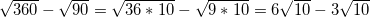Now, with square roots, you can combine factors just as if a given root were a variable. So, just as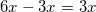, so too does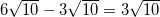### Example Question #5 : How To Subtract Square Roots

Simplify the following: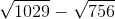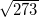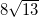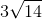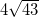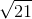Explanation:

Begin simplifying by breaking apart the square roots in question. Thus, you know: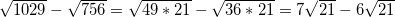Now, with square roots, you can combine factors just as if a given root were a variable. So, just as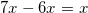, so too does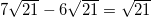### Example Question #6 : How To Subtract Square Roots

Simplify the following: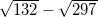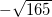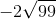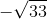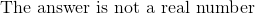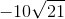Explanation:

Begin simplifying by breaking apart the square roots in question. Thus, you know: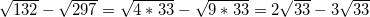Now, with square roots, you can combine factors just as if a given root were a variable. So, just as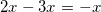, so too does